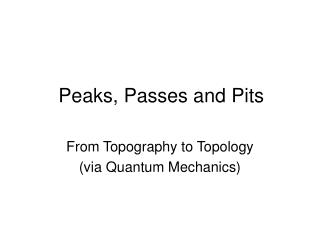DownloadDownload PresentationPeaks, Passes and Pits

# Peaks, Passes and Pits

Télécharger la présentation## Peaks, Passes and Pits

- - - - - - - - - - - - - - - - - - - - - - - - - - - E N D - - - - - - - - - - - - - - - - - - - - - - - - - - -
##### Presentation Transcript

1. Peaks, Passes and Pits From Topography to Topology (via Quantum Mechanics)

2. James Clerk Maxwell, 1831-1879

3. 1861 – “On Physical Lines of Force” • 1864 – “On the Dynamical Theory of the Electromagnetic Field” • 1870 – “On Hills and Dales” • hilldale.pdf

4. Scottish examples

5. Peak Pass Pit

6. Critical points of a function on a surface • Peaks (local maxima) • Passes (saddle points) • Pits (local minima) • Can identify by looking at 2nd derivative • “Topology only changes when we pass through a critical point”.

7. Basic theorem • (# Peaks) – (# Passes) + (# Pits) = Euler Characteristic (V-E+F) • Euler Characteristic is a topological invariant; 2 for the sphere; 0 for the torus. • Does not depend on which Morse function we choose!

8. The Hodge equations • The Euler characteristic can also be obtained by counting solutions to certain partial differential equations – the “Hodge equations”. • They are geometrical analogs of Maxwell’s equations! • To see how PDE can relate to topology, think about vector fields and potentials…

9. The physics connection Ed Witten, Supersymmetry and Morse Theory, 1982

10. Witten’s method • Consider the Hodge equation as a quantum mechanical Hamiltonian. • Different types of ‘particle’ according to the Morse index (‘peakons, passons and pitons’). • Euler characteristic given by counting the low energy states of these particles.

11. Perturbation theory • Replace d by eshd e-sh, where h is the Morse function and s is a real parameter. • This perturbation does not change the number of low energy states. • But it does change the Hodge equations!

12. In fact, it introduces a potential term, which forces our particles to congregate near the critical points of appropriate index. • The potential is s2|h|2 + sXh where Xh is a zero order vectorial term.

13. The term Xh has a ‘zero point energy’ effect which forces each type of particle to congregate near the critical points of the appropriate index; ‘peakons’ near peaks, ‘passons’ near passes and so on.

14. Thus the number of low energy n-on modes approaches the number of critical points of index n, as the parameter s becomes large. • Appropriately formulated, this proves the fundamental result of Morse theory; peaks – passes + pits = Euler characteristic.

15. James Clerk Maxwell, 1831-1879